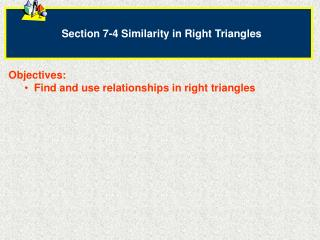Download PresentationSection 7-4 Similarity in Right Triangles

# Section 7-4 Similarity in Right Triangles - PowerPoint PPT PresentationDownload Presentation## Section 7-4 Similarity in Right Triangles

- - - - - - - - - - - - - - - - - - - - - - - - - - - E N D - - - - - - - - - - - - - - - - - - - - - - - - - - -
##### Presentation Transcript

1. Section 7-4 Similarity in Right Triangles • Objectives: • Find and use relationships in right triangles

2. Geometric Mean If you drop a perpendicular from the right angle of a right triangle to the opposite side, you will have three similar right triangles. This altitude is known as the geometric mean. Theorem 7-3The altitude to the hypotenuse of a right triangle divides the triangle into two triangles that are similar to the original triangle and to each other. Right Angle Geometric mean (altitude)

3. Geometric Mean Formula Corollary 1 to Theorem 7-3The length of the altitude to the hypotenuse of a right triangle is the geometric mean of the lengths of the segment of the hypotenuse. x y a b

4. Finding the Geometric Mean For any two positive numbers a and b, the geometric mean of a and b is the positive number y such that, y a b Do Now Find the geometric mean of 4 and 18.

5. Corollary 2 to Theorem 7-3The altitude of the hypotenuse of a right triangle separates the hypotenuse so that the length of each leg of the triangle is the geometric mean of the length of the adjacent hypotenuse segment and the length of the hypotenuse. x y a b

6. Real-World ConnectionThe 300m path to the information center and the 400m path to the canoe rental dock meet at a right angle at the parking lot. Marla walks straight from the parking lot to the lake as shown. How far is Marla from the information center? Hint: Use Corollary 2 Solve for AD AD = 180 Marla is 180m from the info center

7. Explore the Geometric Mean